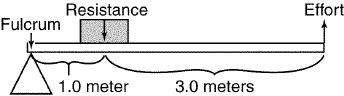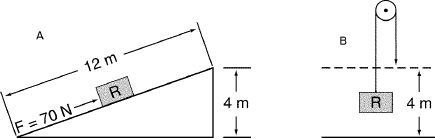Name:    Physical Science Study Guide Chapter 5

 CompletionComplete each statement. 1. Work, like energy, is measured in ____________________. 2. When a machine is used to perform a task, work output is always ____________________ than work input. 3. To calculate mechanical advantage, you divide the ____________________ force by the effort force. 4. Unlike a fixed pulley, a movable pulley ____________________ the effort force. 5. Doorknobs and faucet handles are examples of a simple machine called a ____________________. 6. ____________________ is a measure of how much of the work put into a machine is changed to useful work put out by the machine. Short Answer 7. Which of the following could be the mechanical advantage of a third–class lever: 0.7, 1.5, 10.5, or 3.0? 8. An inventor claims to have built a machine that can produce 120 J of work with an input of 110 J. Would you believe the inventor's claim? Why or why not? 9. Tin snips, designed for cutting metal, have long handles and short blades. Shears designed for cutting paper have short handles and long blades. Explain why this is so. 10. What happens to energy when work is done? 11. Explain why the mechanical advantage of a single fixed pulley is always 1. 12. How are work, time, and power related? 13. Explain why adding oil to the moving parts of a machine can increase its efficiency. 14. Gears are modified wheel-and-axle machines. Explain how to calculate the mechanical advantage of a pair of gears. 15. Sally and Pete do the same amount of work. Sally does the work in 2.3 hours and Pete does it in 2.5 hours. Who is more powerful? Explain. 16. Why is actual mechanical advantage less than ideal mechanical advantage? 17. What is the unit of efficiency? ProblemFigure 5-2 18. Find the ideal mechanical advantage of the second-class lever shown in Figure 5-2. 19. What would be the ideal mechanical advantage of the lever in Figure 5-2 if the fulcrum were moved 2 m to the right?Figure 5-3 20. In Figure 5-3A, what is the ideal mechanical advantage of the inclined plane? 21. In Figure 5-3A, what is the weight of the block? 22. In Figure 5-3A, what is the amount of work required to move the block along the total length of the inclined plane? 23. If the weight of the block in Figure 5-3A were doubled, how much work would be required to move the block along the total length of the inclined plane? 24. If the efficiency of the fixed pulley in Figure 5-3B were 100 percent, how much force would be required in using the pulley to lift the block off the ground? 25. Calculate the ideal mechanical advantage of a lever that is 6 m long and that has 4.5 m effort arm. 26. A 24 cm long screwdriver is used as a lever to open a can of paint. If the fulcrum is 0.5 cm away from the end of the screwdriver, what is the screwdriver's ideal mechanical advantage? 27. Make a sketch of a possible block-and-tackle arrangement that has a mechanical advantage of 4.